Time Travel Research Center © 1998 Cetin BAL - GSM:+90  05366063183 - Turkey/Denizli

Gregory HODOWANEC

Rhysmonic Cosmology

Rhysmonic "Field" Interactions

(3-24-1987)

I. Rhysmonic "Field" Interactions

Figure 1 ---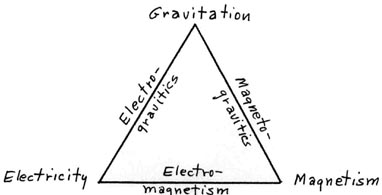A. Premises

(1)   There are but three long-range rhysmonic "field" effects and these are more commonly known as the electrical, magnetic, and gravitational "forces" of physics. There are also two short-range aspects, known as the nuclear forces of physics, but these will not be considered here.

(2)   The relationship between these three aspects of the long-range rhysmonic forces can be depicted as shown in Figure (1). There, electricity and magnetism are shown as forming the base of a triangle formed by these forces since these fields are fairly well understood and their vector field interactions (as electromagnetic effects) are also fairly well understood. However, rhysmonics shows that even here there are some aspects which are not well understood (or even recognized) by the conventional scientist. Gravitation is a more fundamental aspect (and thus shown as the apex of this triangle of forces), but it is also not well understood by conventional science. The scalar aspects of electric and magnetic fields is even less understood by the conventional scientist, while the scalar interaction of gravity with the scalar electric field and the scalar magnetic field is not even recognized by most conventional scientists. 20th century science and technology was largely based on electromagnetism, but 21st century science will include electrogravitic and magnetogravitic interactions, and thus will create much new technology which may even surpass the present day electromagnetic technology.

(3)   The scalar fields (potentials) can add or subtract algebraically, even in the directed vector form, such as the earth’s gravity, provided the components lie in the same direction. For example, the earth’s static electric field and gravitational field are both directed toward the center of the earth. In a small region near the earth’s surface the fields may be considered parallel fields having a vertical orientation. Therefore, these fields can interact with locally created scalar fields and thus lead to new processes, energy sources, as well as levitation, or anti-gravity mechanisms. Simple experiments which illustrate these various interactions will now be briefly considered.

II. Scalar Fields Simplified

Figure 2 ---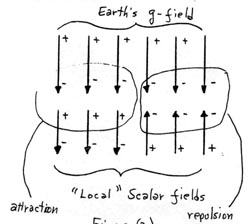Figure 3 ---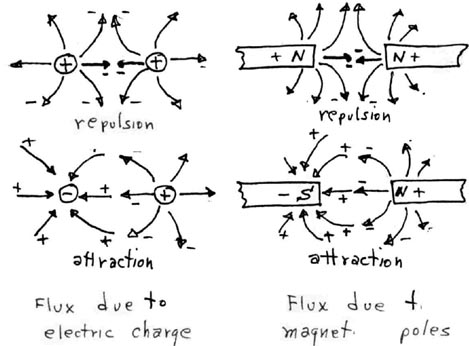A. Premises

(1)   Scalar "flux" lines in rhysmonics are directed rhysmonic vectors and have a reality since they are dynamic effects in the rhysmonic structure which makes up this universe. They are similar to the "lines of force" imagined by Faraday and Maxwell, and are much the same as depicted in some conventional electric and magnetic theory, e.g., the electrostatic and magnetostatic flux lines. Therefore, the interaction of gravitational flux with local scalar flux as shown in Figure (2) is very similar to the interaction of electric and magnetic flux lines as shown in Figure (3), since they are really the same entities. Thus g-fields, E-fields, and H-fields are but different aspects of rhysmonic flux fields.

(2)   The pure scalar field structure of the universe is not directly "observable" since that structure exists as a "perfectly balanced" structure, with the rhysmonic vectors "canceling" each other’s energies. However, the scalar flux gradients as shown in Figure (2) and Figure (3) are "observable" as scalar interaction with matter when observed with the proper instrumentation. For example, the scalar flux due to the earth’s g-field is simply observed as the presence of "weight" in a given mass, which is accelerated by this scalar flux field. The instrumentation required here is but a simple scale system. However, other interactions may be more subtle and thus require special instrumentation as will be briefly considered in this brief.

(3)   To aid the reader in visualization, the flux gradients shown in Figure (2) and Figure (3) are depicted as flowing from (+) to (-). It would be interesting to ascertain if the earth’s g-field and E-field flux are really one and the same?

(4)   While there are many types of scalar signals developed in this universe (as well as man made), emphasis in this short note will be on only a few of these effects. These will now be illustrated with simple scalar field generation and detection methods.

III. Scalar Field Generation

Figure 4 --- Basic Generation of Scalar Fields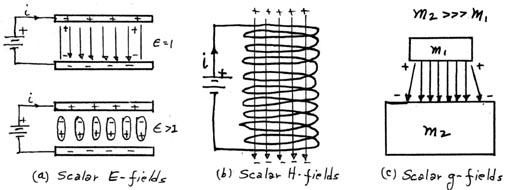A. Premises

(1)   Scalar fields have been shown to be just the electric, magnetic, and gravitational aspects of the basic rhysmonic structure of the universe. Thus their basic generation involves but these three aspects and all scalar field generating systems can be reduced to one of the basic methods shown in Figure (4).

(2)   Scalar E-fields are related in some way to the presence of charge as was shown in Figure (3). A somewhat uniform scalar E-field can be generated by charging the plates of a simple parallel plate capacitor as shown in b. The intensity of this field can be made variable, either by varying the voltage across the plates, the spacing between the plates, or the permitivity of the dielectric material between the plates. In essence, the gradient of the potential is due to an excess of rhysmonic density at the (+) end of the gradient as compared to the (-) end of the gradient.

(3)   Scalar H-fields (curl-free fields) are related to moving charges (mainly electrons) and may be generated by certain permanent magnet configurations, as well as certain current-carrying coil configurations. A simple way of generating scalar H-fields is with a solenoidal coil configuration as shown in Figure (4b). The intensity of this flux is a function of the current and the number of turns in the coils, as well as the coil geometry. An interesting aspect here is that the flux remains constant if the driving voltage is kept constant, but the number of turns in the coil are increased. This results in an apparent paradox in that the input power needed to produce a certain flux decreases as the number of turns, i.e., the wire length increases. This is due to the little realized aspect that the flux generated is a function of the drift velocity of the electrons developing this flux.

(4)   Scalar g-fields are due to the shielding action of masses in this universe as decribed in rhysmonic cosmology. The shielding action creates a net flux in the rhysmonic cosmology. The shielding action creates a net flux in the rhysmonic structure as depicted in Figure (4c). This flux, of course, has been determined by Newton and found to be a function of the masses, the spacing between the masses, as well as the universal rhysmonic structure which is expressed by the gravitational constant, G. The net gravity flux or gradient is also variable with a net change in any of the parameters.

IV. Scalar Field Detection

A. Premises

(1)   Scalar field detection essentially involves the inverse of the generation process shown in Figure (4). The polarization effects in a capacitor as shown in Figure (4a) is a very effective detector of scalar fields of all types, E-, H-, and g-fields, and is briefly discussed in a simple detection system below (Figure 5).

(2)   The solenoidal H-field generator shown in Figure (4b) may also be used in detection in that an incoming scalar flux in tis coil may drive a small electron flow (current) in that coil. While interactions with flux between coils have been used ever since the days of Faraday, and especially by Tesla, the interaction of coils with the gravitational flux will be further considered briefly in the section on magnetogravitic effects.

(3)   The detection of gravitational flux, of course, has always been noted in scale systems of various types. Sensitive Hooke’s Law scale systems have been used as gravimeters for some time now. Here, as depicted in Figure (4c), the action of a gravitational flux on a small mass, m1, due to the shielding action of a much larger mass, m2, which is usually the mass of the earth, may be determined if the small mass is supported by some restoring force (usually a spring). If the restoring spring is made of a compressible conductive foam material, variations in the resistance of the foam will follow the variations in "weight" of the test mass, m1 , which may be due to variations in the earth’s g-fields due top various effects. Such a detector is highly responsive to g-field variations since these affect the scalar fields at the nuclear level, but response is very poor or even non-existent for the scalar E- and H-fields.

(4)   The capacitor element of Figure (4a) (or any other device which can develop polarization "currents" with scalar field interaction), may be used in the very simple detection system shown in Figure (5) below. The unit operates primarily as an electrogravitic device. Here, a scalar flux may "charge" the capacitor to some averaged dc value which may also have ac variations superimposed due to fluctuations in the scalar flux. Thus, this detector responds to all three variations in the scalar fields, but has been especially useful in simple gravitational signal astronomy systems.

Figure 5 --- Simple GW Detection Circuit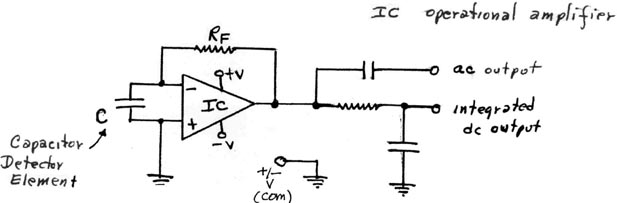V.    Simple Electrogravitic Experiments

A.    Interactions of charge with the Earth’s g-field (or E-field?)

Figure 6 ---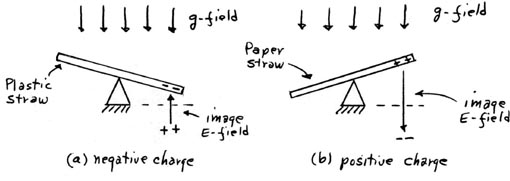(1)   Balance an uncharged plastic soda straw on some fulcrum (A pin pushed through the straw slightly above the centerline axis and supported on a U-shaped bracket is suitable).

(2)   Charge one end of the soda straw negatively by rubbing with dry hands or a cloth. The electric field (E-field) formed by that negative charge and its positive "image" charge will interact with the earth’s g-field (in opposition) and thus the negative end of the straw will be repulsed toward the ground.

(3)   Repeat the experiment with a paper soda straw. The end will now charge positively, and the image field will be reversed and thus attracted by the g-fields and thus will rise; it will be levitated.

B.   Interaction of a parallel plate air capacitor with the Earth’s g-field.

Figure 7 ---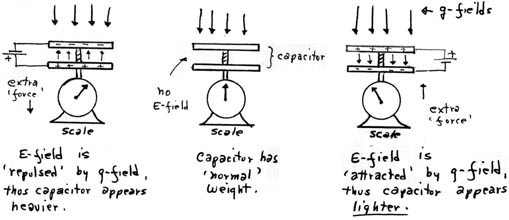Notes: The scale should be a very sensitive Hooke’s Law type. This effect was first demonstrated as the Biefeld-Brown effect many years ago but unfortunately it has largely been ignored!

C.    Universe interactions with GW Detector of Figure 5

Figure 8 ---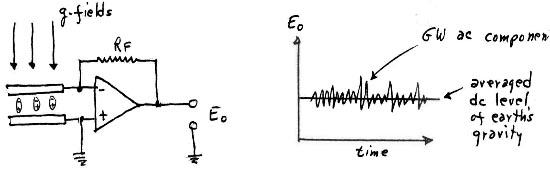(1)   Without using integration in the output, the GW detector will show an averaged dc output which reflects the level of the earth’s gravity field and also an ac component which reflects many oscillatory gravitational processes in the universe.

D.    "Local" interactions with the GW Detector of Figure 5

Figure 9 ---(1)   When the switch, SW, is open (no voltage supplied to the parallel plate air capacitor sandwiching the detector element capacitor, C1, the conditions shown in section C above will prevail.

(2)   When SW is closed, an E-field as shown is created. This field will sum with the g-field, and the detector will read an increase in g-field, or an apparent increase in the earth’s gravity.

(3)   When the parallel plate capacitor voltage is reversed, the opposite conditions will prevail, and the g-field is reduced, for an apparent reduction in the earth’s gravity.

E.    Remarks

There are many possible electrogravitic interactions. For example, energy may be extracted from the earth’s gravity field simply by having this field "charge" a bank of high capacity electrolytic or other capacitors. The above few examples should illustrate to the knowledgeable experimenter the many possibilities.

VI.    Simple Magnetogravitic Experiments

A.   Interaction of Magnets with the Earth’s g-field (or E-field?)

Figure 10 ---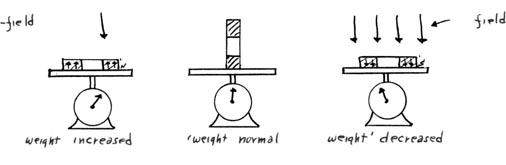(1)    A strongly magnetized ceramic ring magnet having about ¾" OD, ¼" ID, and ¼" thickness is weighed as shown above on a sensitive, non-magnetic Hooke’s Law type scale.

(2)   H-fields (and B-fields) are as shown above. When the H-fields are in the opposite direction from the g-fields, there is "repulsion" and the magnet appears to have an increase in weight. When the H-fields are in the same direction as the g-fields, there is "attraction" and the magnet appears to have a reduction in weight, i.e., there is what appears to be a levitation.

B.    "Local" interactions with the GW Detector of Figure 5

Figure 11 ---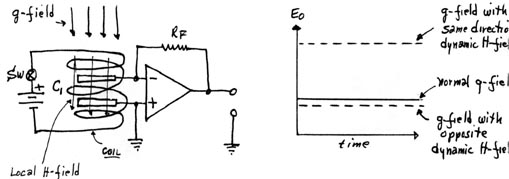(1)   For static H-fields generated by the coil: when the H-fields are in the same direction as the g-fields, a slight increase in the apparent earth gravity field is noticed. When the H-field is reversed, the effect seen is even less.

(2)   For dynamic H-fields generated by the coil: when the collapsing H-fields are in the same direction as the g-fields, the apparent earth gravity field is increased tremendously. With a reversed collapse direction, very little effect is seen on the apparent g-fields.

(3)  This effect will be shown to be of great importance as a future new energy source.

C. Interaction of a Coil with the Earth’s G-Field (or E-Field?)

Figure 12 ---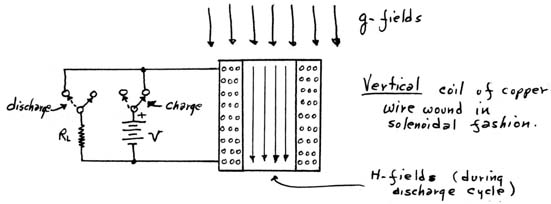Premises

(1) When a voltage is applied to a very long wire solenoid as shown in Figure (12), only a small current flows due to the high resistance of this coil. (as per Ohms Law). However, the magnetic flux developed by such a coil can be very large due to the increased drift velocities of the electrons in the coil under such conditions. The magnetic field is a function of the drift velocity of the electrons as was mentioned in section III-A-3. When the voltage which sustains this drift velocity, and thus the magnetic stress in the vacuum, is suddenly cut of, the energy which was stored in the "magnetic stress" in the vacuum is returned to the circuit as a driving emf and will appear as a high voltage across the coil. In addition, some g-field energy (that was "displaced" from the solenoid by the initial "charging" of the magnetic stress field by the current flow in the solenoid) is also returned to the solenoid. Thus at least two times the initial energy supplied to the solenoid is returned: the original energy that was supplied, plus the energy which is essentially "extracted" from the gravitational field of the Earth! In actual tests, proper control of the experiment has shown that the energy "extracted" by this type of process can be many times the original expenditures!

(2) Such interactions have been demonstrated by many classical physicists in the past. Faraday and others have demonstrated the effects of inductors having indications of such properties. Notable among the classical experimenters was Nikola Tesla, who was a pioneer in demonstrating such effects with his "magnifying transformer" and also in his Tesla coils. Some modern experimenters, including the writer, have also demonstrated many such type reactions. Highly publicized demonstrations have been made by Joe Newman due to his patent problems. However, while Newman’s demonstrations are valid, they are the result of the above effects and not any extraction of energy from mass as he claims, at least in the writer’s opinion.

(3) That the above is a more correct description of the energy extraction mechanism is simply demonstrable in the experiments shown in section VI-B. It is also significant that the coils in most such experiments must be in a vertical position for most effectiveness.

(4) The following experiment also emphasizes the aspect in (3), mentioned above.

VII. Simple Electro-Magneto-Gravitic Experiment

Figure 13 ---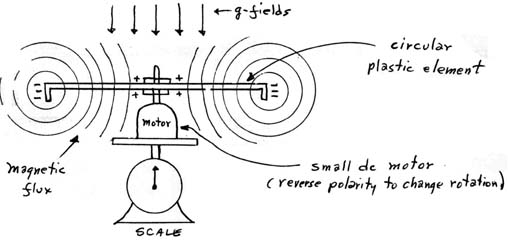This very simple experiment demonstrates the interaction of all three long range "forces" in this universe. These aspects have been noted by several classical period researchers in the past as well as some more recent experimenters, e.g., John Searl of England, but their efforts have been largely ignored.

Premises

(1) The rim of the plastic element is charged negatively, either by induction or just the spinning action itself. The test assembly is mounted atop a sensitive, non-magnetic Hooke’s Law type of scale.

(2) With a clockwise (CW) rotation of the plastic element, the electrons on the rim are carried around by the rim at high velocities and thus are, in effect, a strong electrical circuiting current which thus creates a very intense magnetic field (H-field) in the central region of the disc. The H-field flux is directed down, in the same direction as the g-fields and thus are "attracted" by the g-fields. Therefore, the rotating element’s weight is apparently reduced.

(3) With counterclockwise (CCW) rotation, the rim "current" is reversed and thus the direction of the H-fields in the center region are now up and thus they oppose the g-fields. Therefore, the element’s "weight" is now apparently increased.

(4)   Such aspects have been noted by many earlier researchers, especially in experiments with electrostatic field generating devices. The more recent applications by researchers such as John Searl have resulted in levitation and high energy "extraction", and thus have been used to possibly explain "flying saucers" in a way. However, these experiments and the explanations were never received kindly by conventional science and thus have been largely ignored and even suppressed! The writer believes that many of these experiments are valid and will eventually lead to new transportation methods. Efforts in these aspects are increasing all the time.

VIII.   Gravitational Signal Astronomy

These aspects have been released by the writer in a number of brief articles, some of which have been published, and many others not yet published. Gravitational signal astronomy has been most productive and some possibly profound effects have been noted, not only affecting our knowledge of the universe, but even the quality of our life here on Earth. Some brief notes on this technique are now presented here:

(1) Gravitational "fluctuations" in the universe are best detected as modulations in the signal output of a GW detector of the type shown in Figure (5). The scalar gravity signals interact with the Earth’s g-fields (or E-fields) which are directed toward the center of the earth. Thus the variations which are located on the observer’s meridian, and especially in his zenith, are best detected. It can be shown that the earth’s rotation effectively cancels response from the other directions.

(2) The beam "width" of the detector is essentially equal to the area of the active portion of the detector element, and thus may be only in the order of a square inch, typically. Thus the resolution of the detector is extreme, much finer than most other detection systems, be it scalar or electromagnetic in nature.

(3)   Detection from directions other than the meridian also are possible. Two examples are given here:

(a) When large masses line up with the observer’s position, e.g., as in a solar eclipse, the gravitational "shadows" will also fall on the observer’s position.

(b) "Stacked Array" GW detection systems could enhance detection in a particular direction. Such arrays may also enhance the detection of objects located on the observer’s meridian but off the zenith. These arrays could be terms "Gravity Signal Telescopes".

IX. Conclusions

The notes contained in this Brief were directed primarily at those colleagues of the writer who have shown interest in the rhysmonic cosmology methodology. The simplicity of the experiments should enable the interested experimenter or researcher to easily verify these various aspects. The researcher may come up with other simple tests, while the writer will disclose many others later on. Meanwhile, good luck with your experimentations!

 Hiçbir yazı/ resim  izinsiz olarak kullanılamaz!!  Telif hakları uyarınca bu bir suçtur..! Tüm hakları Çetin BAL' a aittir. Kaynak gösterilmek şartıyla  siteden alıntı yapılabilir. © 1998 Cetin BAL - GSM:+90  05366063183 -Turkiye/Denizli  Ana Sayfa / Index / Roket bilimi / E-Mail / Kuantum Fizigi / Astronomy                                        / Time Travel Technology /UFO Galerisi / UFO Technology/